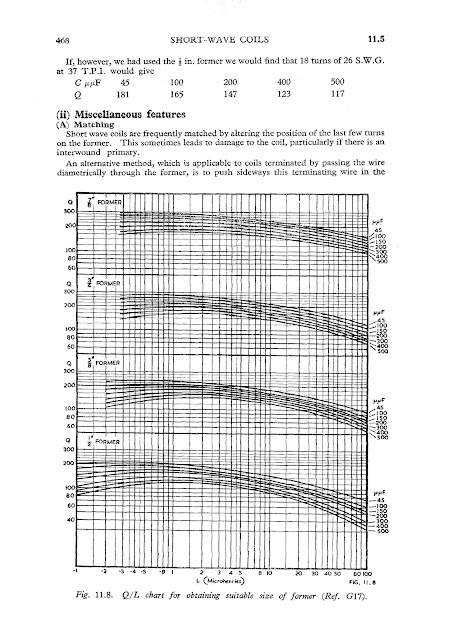## Friday, February 26, 2016

### The square coil

The coil inductance is determined by this formulasThe factor are length of winding and radius of the windings. K is found from a chart.The Diameter to Length ratio is calculated and then the K is found on the chart. The inductance to resistance ratio is the Q so a larger wire with less resistance or a shorter wire of smaller gauge will give different results. For the rule of thumb a 'square' coil will be the best compromise. There are to many variables to just go square though. For example a transmitter coil may be wound with 1/4" copper tubing.This example shows the 'square' coil has more inductance with the same size and number of turns the wire resistance will be the same and the Q will be  higher for the 'square' coil. However if the 'square'coil was a receiver coil wound with 24 guage wire and the other coil was a transmitter coil wound with 1/4" copper tubing it could have a high Q so they could both serve their purpose. You could use this chart to find your values.Then determine the Q you want and use this chart to see some posibilities.The chart shows a larger diameter coil is higher Q.